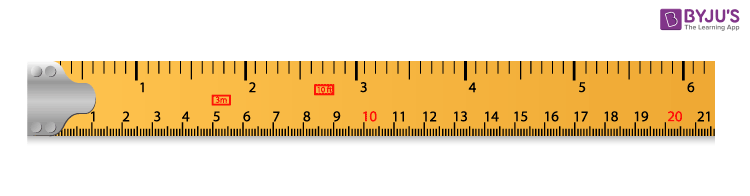Checkout JEE MAINS 2022 Question Paper Analysis : Checkout JEE MAINS 2022 Question Paper Analysis :

# Centimeter

A centimeter is a metric unit for the measurement of length of objects and small distances. It is written using the symbol cm. It can also be defined as the unit of length in the International System of Units (SI), the current form of the metric system. It is equivalent to 1/100 meters.

A centimeter is based on the concept meter, SI unit, and as the prefix “centi” denotes that is equal to one-hundredth of a meter. Based on the decimal system, the range of the metric is the factors from 10-18 to 1018, with the base having no prefix and 1 as the factor. Here, the base is the meter. Also, 1 cm is equivalent to 0.39370 inches.

The three conventional tools of measurement are namely ruler, meter stick and tape.

Ruler: A ruler is the most commonly used geometric tool for measurement in mathematics. It is used to measure the length or height of small things such as notebooks, pens, pencils and so on. Most of the rulers contain two measurement metrics namely centimeters and inches. Also, these rulers have lengths of 15 cm and 30 cm in most of the cases.

Meter Stick: A measurement tool that measures one meter, i.e. 100 centimeters and is used to measure objects in meters and centimeters is called a meter stick. For example, the length of a small rope, height of a table or the width of a room, etc., can be measured with the help of a meter stick.

Tape: A tape (measuring tape) is a flexible ruler that measures the length of objects which are curbative in nature such as ribbons, cloths, rings and so on.

## Centimeter Scale

The scale for centimeters measurement can be easily understood with the help of a ruler. The figure below shows the measurement of length in centimeters.This measurement is used to measure small distances between objects or dimensions of small objects in real-life situations.

### Centimeter Chart

Different charts can be constructed for showing the conversion of units from centimeters to other units of measurement such as meters, inches, feet and so on. Using these charts, one easily remembers the conversion of units from centimeters to other units.

## How many centimeters in one meter

As we know, a centimeter is equivalent to one-hundredth of a meter. Thus, there will be 100 centimeters in one meter.

100 cm = 1 m

Or

1 cm = 1/100 m

### Centimeter to Meters

The conversion chart from cm to m is given below:

 Centimeters (cm) Meters (m) 1 cm 0.01 m 2 cm 0.02 m 5 cm 0.05 m 10 cm 0.1 cm 50 cm 0.5 m 100 cm 1 m 500 cm 5 m 1000 cm 10 m

Also, check the Centimeter to meters conversion tool here.

### Centimeter to Feet

The conversion chart from centimeters (cm) to feet (ft) is given below:

 Centimeters (cm) Feet (ft) 1 0.03281 2 0.06562 3 0.09843 5 0.16404 10 0.32808 20 0.65617 30 0.98425 40 1.31234 50 1.64042 100 3.28084

### Centimeter to Inch

The conversion chart for centimeters (cm) to inches (in or “) is given below:

 Centimetre Inches in Decimal Inches in Fraction 1 cm 0.3937 in 25/64 in 2 cm 0.7874 in 25/32 in 3 cm 1.1811 in 1 (3/16) in 4 cm 1.5748 in 1 (37/64) in 5 cm 1.9685 in 1 (31/32) in 6 cm 2.3622 in 2 (23/64) in 7 cm 2.7559 in 2 (3/4) in 8 cm 3.1496 in 3 (5/32) in 9 cm 3.5433 in 3 (35/64) in 10 cm 3.9370 in 3 (15/16) in

Check: cm to feet conversion

### Centimeter to Millimeters

One centimeter is equivalent to ten millimeters, i.e. 1 cm = 10 mm. The conversion table to understand the conversion from cm to mm is given below:

 Centimeters (cm) Millimeters (mm) 1 cm 10 mm 2 cm 20 mm 5 cm 50 mm 10 cm 100 mm 50 cm 500 mm 100 cm 1000 cm 1000 cm 10000 mm

Get cm to mm converter here.